# Python输入与输出

...PythonPython大约 3 分钟

### # 输入

``````a = input("提示的内容：")
print(a) # 后面会讲这里
``````

### # 输出

#### # 基本的输出

``````print("Hello heStudio!")
``````

#### # 输出变量

``````a = "jntm"
print(a)
``````

#### # 分清变量和字符串的区别

``````>>> a = jntm
>>> print(a)
jntm
``````
``````>>> print("a")
a

``````

#### # 将`print()`里的多项连接

##### # 使用`, `连接
``````time = "两年半"
print("我是练习时长", time, "的练习生")
``````

##### # 使用`+`连接
``````c = "只因"
print(c+"你太美")
``````

##### # 或者混合使用
``````print("唱"+"跳","rap","篮球")
``````

### # 输出多行文字

``````print("""
1
2
3
""")
``````

#### # 简单的数据类型转换

`int()`整型
`float()`浮点型
`str()`字符串
`repr()`字符串

`str()``repr()` 的区别 `str()`主要用来为终端用户输出一些信息，而`repr()`主要用来调试

``````a = int("1")
b = int("2")
print(a+b)
``````

#### # 转义符号

`\\`输出反斜杠
`\'`输出单引号
`\"`输出双引号
`\n`换行
`\t`水平制表符
`\r`光标回到首位
`\b`退格

``````print(r"Hello\nWorld")
``````

### # 学以致用

#### # 简单加法计算器

``````a = float(input("请输入第一个加数："))
b = float(input("请输入第二个加数："))
print(str(a),"+",str(b),"=",float(a+b),"\n计算完毕！")
``````

1. 一个制表符的作用就是重新开一个新的制表位，一个制表位的长度为4个字符。如果之前的字符占满了上一个制表位，就重开一个，若果没有，则是把之前的一个制表位给填充满。 ↩︎

•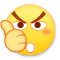0
•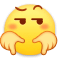0
•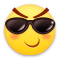0
•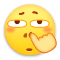0
•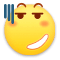0
•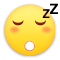0

• 按正序
• 按倒序
• 按热度